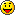SGDK only sub forum

Moderator: Stef

danibus
Very interested
Posts: 72
Joined: Sat Feb 03, 2018 12:41 pm

Just a simple question about sinFix16(value)

I'm working with a sprite with horizontal movement, always to the left, then this sprite will disappear from left side of screen and then appear on the right side... and so. I wanted a sin movement, then made:

y = 84 + sinFix16(x); // 84 is the initial 'y' position of the sprite

sinFix16(x) goes from 64 to -64, so Y = 20 .. 148

Why 64 to -64 ???? I read that sinFix16(value) needs a value from 0 to 1024, I just put 'x' and works... not sure whyI just working with int
If I want to a better sin movement, use fix32 or fix16?

danibus
Very interested
Posts: 72
Joined: Sat Feb 03, 2018 12:41 pm

Code: Select all

``````static void moveEnemy()
{
int vmovx = 1;

enemyPosx -= vmovx ;		// move to the left, enemyPosx  and enemyPosy    are INTs
enemyPosx = contsY + sinFix16(enemyPosx);   // contsY =84;

SPR_setPosition(enemy, enemyPosx, enemyPosy);

}
``````
This is my function to move enemy sprite with sinusoidal movement.
I want sprite to move up/down across the screen, but it moves to slow to do it only in one screen.

ThisI tried

Code: Select all

``enemyPosx = contsY + sinFix16(enemyPosx) * x;  // x = 1.25   or  2``
but also to fast going up/down

Maybe using INTs for (x,y) is the problem

Chilly Willy
Very interested
Posts: 2799
Joined: Fri Aug 17, 2007 9:33 pm

danibus wrote:
Fri Aug 02, 2019 3:42 am
Why 64 to -64 ???? I read that sinFix16(value) needs a value from 0 to 1024, I just put 'x' and works... not sure whyIt goes from 64 to -64 because with the fixed point 16-bit numbers in SGDK, that's 1 to -1. Remember, the fractional part of the fix16 is 6 bits. The table goes from 0 to 1024 because it's a binary angle. Binary angles use convenient powers of two so that you can use AND to clamp the range to the table. Radians are not so good a unit for a processor like the 68000.1024 entries gives a sufficiently smooth sine wave without taking too much rom space. Especially considering the limits of the fractional parts of fixed point numbers (fix16 in particular).

Stef
Very interested
Posts: 2993
Joined: Thu Nov 30, 2006 9:46 pm
Location: France - Sevres
Contact:

Chilly Willy perfectly explained the reason of the value you obtained and sin table sizeIf you want faster sin wav depending x position, try something as :

Code: Select all

``enemyPosy = contsY + sinFix16(enemyPosx * sinSpeed);``
If you also want larger sin amplitude then multiply that way :

Code: Select all

``enemyPosy = contsY + sinFix16(enemyPosx * sinSpeed) * Amplitude;``

danibus
Very interested
Posts: 72
Joined: Sat Feb 03, 2018 12:41 pm

Thanks for replying. Not sure I understand hehe

2 ^ 6 = 64 (fractional part of the fix16 is 6 bits)

So let's check

Code: Select all

``````...
int enemyPosx = 260;  //starter position
...
int sinSpeed = 2;
int Amplitude = 1;
enemyPosx = enemyPosx - 1;   //moves 1 pixel (60 pixels/sec aprox)
enemyPosy = contsY + sinFix16(enemyPosx * sinSpeed) * Amplitude;
...
``````
Enemy is moving from right to left:

enemyPosy = 84 + sinFix16(260 * 2) * 1 = 84 + sinFix16(520) = 81 ( then sinFix16(520) = -3 )
enemyPosy = 84 + sinFix16(259 * 2) * 1 = 84 + sinFix16(518) = 82 ( then sinFix16(518) = -2 )
enemyPosy = 84 + sinFix16(258 * 2) * 1 = 84 + sinFix16(516) = 82 ( then sinFix16(516) = -2 )
enemyPosy = 84 + sinFix16(257 * 2) * 1 = 84 + sinFix16(514) = 83 ( then sinFix16(514) = -1 )
enemyPosy = 84 + sinFix16(256 * 2) * 1 = 84 + sinFix16(512) = 84 ( then sinFix16(512) = 0 )
...

SINE WAVE table have 1024 entries ( varies 2 every time):
1024 -> 0 ---> that means sinFix16(1024) = 0
1022 -> -1
1020 -> -2
1018 -> -2
1016 -> -3
...
768 -> -64
...
516 -> -2
514 -> -1
512 -> 0
510 -> 1
508 -> 2
...
2 -> 1
0 -> 0

That means starts with negative values (SIN wave starts moving down... then goes up to 0, goes up and goes down again)
from 0 to -64, from -64 to 0 to 64, again from 64 to 0

IF Amplitude = 2, then sinFix16() will go from 0 to -128, from -128 to 0 to 128, again from 128 to 0
And so

Chilly Willy
Very interested
Posts: 2799
Joined: Fri Aug 17, 2007 9:33 pm